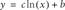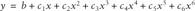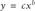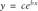# Generating Nonlinear Curve Fits Using Excel Charts

Problem

You'd like to generate best-fit curvesnonlinear curvesthrough your data.

Solution

Use Excel's chart trendline feature to perform nonlinear curve fits of your data. Plot your data using an XY scatter chart (see Chapter 4). Once your chart has been created, right-click on the data series and select Add Trendline from the pop-up menu.

Discussion

The procedure for adding nonlinear trendlines to charts is the same as that used for adding linear trendlines, as discussed in Recipe 8.1. Once you've plotted your data using an XY scatter chart, you can generate a trendline, which will be displayed on the chart, superimposed over your data. You can also include the resulting equation for the best-fit line on your chart.

When you add a trendline using the procedure discussed in Recipe 8.1, you can choose from several different Trend/Regression types (as shown in the Add Trendline dialog box in Figure 8-1). Depending on the range of values in your data, you'll be able to choose from six different trendlines. Of these six, four are nonlinear trendlines: Logarithmic, Polynomial, Power, and Exponential. The other two include the Linear trendline already discussed and a Moving Average trendline.

The Logarithmic trendline uses the equation:where c and b are parameters to be determined during the curve-fitting process.

Polynomial trendlines use the equation:where the c-coefficients and b are parameters of the curve fit. Excel supports polynomial fits up to sixth order. You can select the order to use in the Add Trendline dialog box (See Figure 8-1).

Excel's Power trendline uses the equation:where c and b are parameters of the fit.

Finally, the Exponential trendline uses the equation:where c and b are parameters of the fit.

The natural question that arises upon learning of these available models is which one to use. There's no single answer to this question, as it depends on your purpose for performing the fit and the nature of your data. If you're simply fitting a curve through some data so you can conveniently interpolate the data, then choose whichever model best replicates your data. In this case, you're not so concerned with smoothing the data or with statistical rigor. To assess which model works, you can look at the R-squared value (see Recipe 8.7) or you can eyeball the trendline against your data. Further, you can plot the residualsdifferences between your actual y-value and the estimated y-valueand examine them to assess how well your data is represented. You can compute percentage differences to gauge how well your data is replicated. Further, you should examine how the trendline behaves between data points used to generate the trendline to make sure there are no unrealistic oscillations between data points. This can happen when you try to fit a higher-order polynomial trendline.

On the other hand, if you're genuinely interested in modeling the data from a statistical standpoint, or are trying to gain insight in a physical process, then I suggest you read Recipe 8.7. Further, you must also consider what your data represents. If your data represents some physical process, then let physics be your guide and choose a model that best represents the physical relationship between the variables underlying the data, if it is indeed known. If none of Excel's trendline models work for you, then you're left to your own devices to craft a suitable model. In this case, take a look at Recipe 8.6.

Take a look at Recipes 8.6 and 8.7. Also, be sure to read Recipe 8.1 for a refresher on adding trendlines to charts.Excel Scientific and Engineering Cookbook (Cookbooks (OReilly))
ISBN: 0596008791
EAN: 2147483647
Year: N/A
Pages: 206
Authors: David M Bourg